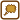## Capillary with a Sealed End

A glass capillary with inner diameter of d = 0.2 mm and length l = 20 cm is sealed at one end. The other end is submerged in water in a way that the water surface inside and outside are in the same height. The capillary’s end is h = 2.9 mm deep. How big is the surface tension of water against air?

• #### List of Known Information

 r = 0.1 mm = 10−4 m capillary’s inner diameter l = 20 cm = 2·10−1 m length of the capillary h = 2.9 mm = 2.9·10−3 m length of submerged part of the capillary pa = 105 Pa atmospheric pressure σ = ? water’s surface tension
• #### Analysis

First think through what happens to the air inside the capillary when we submerge it in water. The air fills the capillary and has atmospheric pressure in the beginning. After submersion water gets inside the capillary and the volume of the air decreases which causes a rise in its pressure. This rise is the same as the capillary pressure.

We can consider air to be ideal gas at normal conditions. During the submersion the temperature of the air will not change. We can write the equation of state for isothermal process for the air before and after the submersion. We will then express pressure from the equation. The capillary pressure can also be expressed using the radius of the capillary and the wanted surface tension of water.

• #### Hint 1 - pressure and volume of air inside the capillary

Think through what the volume and pressure of air inside the capillary before and after the submersion is.

• #### Hint 2 - equation of state

The air inside the capillary can be considered an ideal gas at normal conditions. Write the equation of state for air inside the capillary before and after submersion. What process can we consider the air compression to be?

• #### Hint 3 - relation for capillary pressure calculation

Recall the relation for capillary pressure calculation. The capillary pressure can also be expressed from the equation of state. We will then determine the water surface tension from these two equations.

• #### Hint 3 solution - relation for capillary pressure calculation

This equation applies for capillary pressure pk

$p_\mathrm{k} = \frac{2σ}{r},\tag{6}$

where σ is the surface tension of a liquid and r is the radius of the capillary.

• #### Complete Solution

The pressure inside the capillary is the atmospheric pressure pa before we submerge the capillary into water.

The volume of the capillary V1 can be calculated as

$V_\mathrm{1} = πr^{2}l,\tag{1}$

where r is the diameter of the capillary and l is the length of the inner side of the capillary.

The product of the pressure and the air volume inside the capillary before submersion is

$p_\mathrm{1}V_\mathrm{1} = p_\mathrm{a}πr^{2}l.$

The volume of air will decrease to V2 after the submersion of the capillary into the water

$V_\mathrm{2} = πr^{2}(l−h),\tag{2}$

where h is the height of water inside the capillary.

The air pressure inside the capillary will also increase by the capillary water pressure pk. The pressure in the capillary will then be p2

$p_\mathrm{2} = p_\mathrm{a} + p_\mathrm{k}.\tag{3}$

After the submersion, the product of the pressure and the air volume in the capillary will be equal to

$p_\mathrm{2}V_\mathrm{2} = πr^{2}(p_\mathrm{a} + p_\mathrm{k})(l−h).$

According to the equation of state for isothermal process, this applies

$p_\mathrm{1}V_\mathrm{1} = p_\mathrm{2}V_\mathrm{2}\tag{4}$

after substitution from equations (1), (2) and (3) into the equation of state, we receive

$p_\mathrm{a}πr^{2}l = πr^{2}(p_\mathrm{a} + p_\mathrm{k})(l−h).\tag{5}$

We will adjust the relation and we will express the capillary pressure

$p_\mathrm{k} = \frac{p_\mathrm{a}h}{l−h}$

We want to know the value of the surface tension of water. We can calculate the surface tension σ using the value of the capillary pressure and relation

$p_\mathrm{k} = \frac{2σ}{r}.\tag{6}$

Substituting the relation (6) into adjusted relation (5) will yield us relation for calculation of the surface tension of water

$σ = \frac{p_\mathrm{a}rh}{2(l−h)}.\tag{7}$
• #### Numeric Substitution

The value of surface tension of water can be calculated from relation (7)

$σ = \frac{p_\mathrm{a}rh}{2(l−h)}.$

After substitution of numeric values from assignment, we receive equation

$σ = \frac{10^{5}·10^{−4}·2.9·10^{−3}}{2·(2·10^{−1}−2{,}9·10^{−3})} N·m^{−1}$ $σ \dot{=}\, 74·10^{−3} N·m^{−1}.$